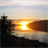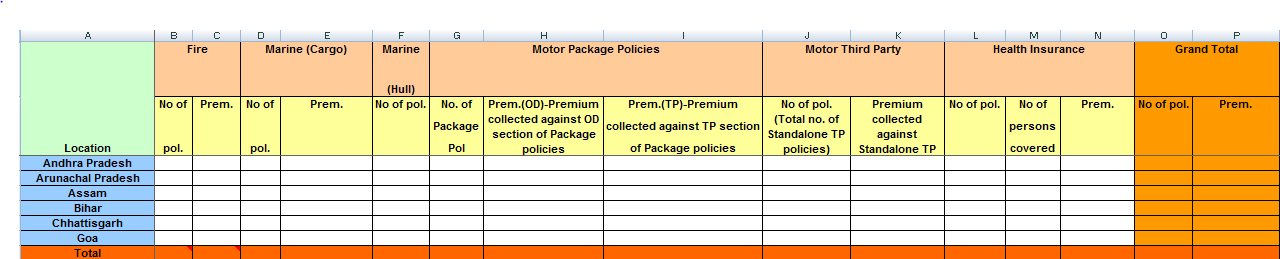# QlikView App Dev

Discussion Board for collaboration related to QlikView App Development.

Announcements
Customer & Partners, DEC. 9, 11 AM ET: Qlik Product & Strategy Roadmap Session: Data Analytics REGISTER NOW
cancel
Showing results for
Did you mean:Partner

## Summarize Total Issue on a Calculated Dimension

Dear Qlik Guys,

Greetings...!!!

We have a below requirement where we have 3 dimensions out of which 1 is derived using Inline. we need each dimension to calculate No. of pol & Prem amnt but for certain dimensions we need to calculate No. of pol, od prem amnt & tp prem amnt.

PFB requirement:I have used below expression to map my different dimensions.

=Pick(Match(VALUE,      'No of persons covered',

'No of pol.',

'No of pol.(Total no. of Standalone TP policies)',

'No. of Package Pol',

'Prem.',

'Prem.(OD)-Premium collected against OD section of Package policies',

'Prem.(TP)-Premium collected against TP section of Package policies',

'Premium collected against Standalone TP policies'),

Num((Sum({<Freeze_Date={">=\$(vQuarterStart)<=\$(vQuarterEnd)"},FiscalYearDesc=,FiscalQuarter=,Month=,POL_ENDORSEMENT_TYPE = {'POLICY'}, DATA_FETCH_FLAG -= {'TOTAL LOSS'},  POL_INSURANCE_TYPE -= {'REINSURANCE'}, POL_DATA_FLAG-={'T','A','R','VIRTUAL'},MTD_FLAG={'Y'} >}POL_TOT_LIVES_INSURED)), '#,##0'),

Num((Sum({<Freeze_Date={">=\$(vQuarterStart)<=\$(vQuarterEnd)"},FiscalYearDesc=,FiscalQuarter=,Month=,POL_ENDORSEMENT_TYPE = {'POLICY'}, DATA_FETCH_FLAG -= {'TOTAL LOSS'},  POL_INSURANCE_TYPE -= {'REINSURANCE'}, POL_DATA_FLAG-={'T','A','R','VIRTUAL'},MTD_FLAG={'Y'} >}POLICY_COUNT)),'#,##0'),

Num((Sum({<Freeze_Date={">=\$(vQuarterStart)<=\$(vQuarterEnd)"},FiscalYearDesc=,Month=,FiscalQuarter=,

POL_INSURANCE_TYPE -= {'REINSURANCE'}, POL_ENDORSEMENT_TYPE = {'POLICY'}, DATA_FETCH_FLAG -= {'TOTAL LOSS'},TP_FLAG = {'Y'},  POL_DATA_FLAG -={'T','A','R','VIRTUAL'},MTD_FLAG={'Y'} >}POLICY_COUNT)),'#,##0'),

Num((Sum({<Freeze_Date={">=\$(vQuarterStart)<=\$(vQuarterEnd)"},FiscalYearDesc=,Month=,FiscalQuarter=,

POL_INSURANCE_TYPE -= {'REINSURANCE'}, POL_ENDORSEMENT_TYPE = {'POLICY'}, DATA_FETCH_FLAG -= {'TOTAL LOSS'},TP_FLAG = {'Y'},POL_DATA_FLAG-={'T','A','R','VIRTUAL'},MTD_FLAG={'Y'}>}POLICY_COUNT)

+ Sum({<Freeze_Date={">=\$(vQuarterStart)<=\$(vQuarterEnd)"},FiscalYearDesc=,Month=,FiscalQuarter=,

POL_INSURANCE_TYPE -= {'REINSURANCE'}, POL_ENDORSEMENT_TYPE = {'POLICY'}, DATA_FETCH_FLAG -= {'TOTAL LOSS'},OD_FLAG = {'Y'},POL_DATA_FLAG-={'T','A','R','VIRTUAL'},MTD_FLAG={'Y'}>}POLICY_COUNT)), '#,##0'),

Num((Sum({<Freeze_Date={">=\$(vQuarterStart)<=\$(vQuarterEnd)"},FiscalYearDesc=,FiscalQuarter=,Month=,

POL_INSURANCE_TYPE -= {'REINSURANCE'},POL_DATA_FLAG-={'T','A','R','VIRTUAL'},MTD_FLAG={'Y'}>} GWP) / vDecimal), '#,##0.00'),

Num((Sum({<Freeze_Date={">=\$(vQuarterStart)<=\$(vQuarterEnd)"},FiscalYearDesc=,FiscalQuarter=,Month=,

POL_INSURANCE_TYPE -= {'REINSURANCE'},POL_DATA_FLAG-={'T','A','R','VIRTUAL'},MTD_FLAG={'Y'},OD_FLAG = {'Y'}>}CALC_OD_OS)/ vDecimal), '#,##0.00'),

Num((Sum({<Freeze_Date={">=\$(vQuarterStart)<=\$(vQuarterEnd)"},FiscalYearDesc=,FiscalQuarter=,Month=,

POL_INSURANCE_TYPE -= {'REINSURANCE'},POL_DATA_FLAG-={'T','A','R','VIRTUAL'},MTD_FLAG={'Y'},OD_FLAG = {'Y'}>}CALC_TP_OS)/ vDecimal), '#,##0.00'),

Num((Sum({<Freeze_Date={">=\$(vQuarterStart)<=\$(vQuarterEnd)"},FiscalYearDesc=,FiscalQuarter=,Month=,

POL_INSURANCE_TYPE -= {'REINSURANCE'},POL_DATA_FLAG-={'T','A','R','VIRTUAL'},MTD_FLAG={'Y'}>}CALC_TP_OS)/ vDecimal), '#,##0.00')

)

I need a final summarized total that is my Grand Total which has to be split into 2 parts i.e No. of Pol & Prem Amnt which is currently not showing as I have only single expression which is defined above. Also same is not possible using Partial Sums.

Kindly assist and provide your valuable inputs which would be of great help.